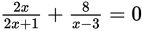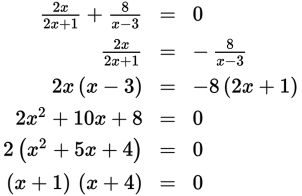# SAT Math Multiple Choice Question 901: Answer and Explanation

### Test Information

Question: 901

1. If a and b are solutions to the equation, and a < b, what is the value of a?

• A. -4
• B. -1
• C. 1
• D. 4

Explanation:

A

Difficulty: Medium

Category: Passport to Advanced Math / Exponents

Strategic Advice: When a rational equation has two terms (not counting 0), move one term to the other side of the equation and cross-multiply.

Getting to the Answer: Move the second term to the right-hand side of the equal sign by subtracting it (mentally) from both sides. Then cross-multiply. The result gives a quadratic equation, which you can solve by factoring:Setting each factor equal to 0 gives x = -1 and x = -4. Neither solution is extraneous, and the question states that a < b, so a is the smaller of the two values, which is -4, or (A).